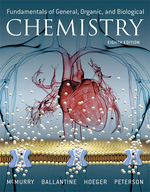×
Get Full Access to Fundamentals Of General, Organic, And Biological Chemistry (Mastering Chemistry) - 8 Edition - Chapter 14.3 - Problem 14.7
Get Full Access to Fundamentals Of General, Organic, And Biological Chemistry (Mastering Chemistry) - 8 Edition - Chapter 14.3 - Problem 14.7

×ISBN: 9780134015187 2044

## Solution for problem 14.7 Chapter 14.3

Fundamentals of General, Organic, and Biological Chemistry (Mastering Chemistry) | 8th Edition

• Textbook Solutions
• 2901 Step-by-step solutions solved by professors and subject experts
• Get 24/7 help from StudySoup virtual teaching assistantsFundamentals of General, Organic, and Biological Chemistry (Mastering Chemistry) | 8th Edition

4 5 1 288 Reviews
14
5
Problem 14.7

For each of the following molecules, (i) redraw using line structure format, (ii) identify its hydrophobic and hydrophilic parts, and (iii) predict its solubility in water.

(a) $$\mathrm{CH}_{3}\left(\mathrm{CH}_{2}\right)_{10} \mathrm{CH}_{2} \mathrm{OH}$$Text Transcription:

CH_3(CH_2)_10CH_2OH

Step-by-Step Solution:
Step 1 of 3

Chapter T wo: Measurement, Problem Solving, and the Mole Concept Thursday, September 1, 2016 8:40 PM 2.1: "The Metric Mix-Up: A \$125 Million Unit Error"  Basically a mars weather monitor failed because they mixed up units.  Units are critical 2.2: "The Reliability of Measurement"  Reliability depends on the instrument used to measure  More digits in measurement = more reliability o Scientists do this on purpose to reflect reliability of instrument o Uncertainty is in the last recorded digit (+/- 1)  Accuracy: how close the measured value is to the actual value  Precision: how close the measured values are to each other or how reproducible they are  Random Error: error that has equal probability of being too high or low o Averages out with repeated trials  Systematic error: tends to be either too high or too low o Doesn't average out. 2.3: "Density"  Density of a substance is the ratio of its mass to its volume (density=mass/volume) o A physical property of a substance o Depends on the temperature of the substance o An intensive property: independent of the amount of the substance  Mass, for example, in an extensive property (depends on the amount of the substance. Only knowing something's mass doesn't identify it.) 2.4: "Energy and Its Units"  Two fundamental components of our universe are matter and energy  Energy: the capacity to do work o Work: th

Step 2 of 3

Step 3 of 3

##### ISBN: 9780134015187

The answer to “?For each of the following molecules, (i) redraw using line structure format, (ii) identify its hydrophobic and hydrophilic parts, and (iii) predict its solubility in water. (a) $$\mathrm{CH}_{3}\left(\mathrm{CH}_{2}\right)_{10} \mathrm{CH}_{2} \mathrm{OH}$$ Text Transcription:CH_3(CH_2)_10CH_2OH” is broken down into a number of easy to follow steps, and 32 words. Since the solution to 14.7 from 14.3 chapter was answered, more than 200 students have viewed the full step-by-step answer. The full step-by-step solution to problem: 14.7 from chapter: 14.3 was answered by Aimee Notetaker, our top Chemistry solution expert on 04/25/22, 03:57PM. This full solution covers the following key subjects: . This expansive textbook survival guide covers 281 chapters, and 456 solutions. Fundamentals of General, Organic, and Biological Chemistry (Mastering Chemistry) was written by Aimee Notetaker and is associated to the ISBN: 9780134015187. This textbook survival guide was created for the textbook: Fundamentals of General, Organic, and Biological Chemistry (Mastering Chemistry), edition: 8.

## Discover and learn what students are asking

Statistics: Informed Decisions Using Data : Applications of the Normal Distribution
?In Problems 19–22, find the value of ?? ?0.025

Statistics: Informed Decisions Using Data : Inference about Two Population Standard Deviations
?In Problems 9–14, assume that the populations are normally distributed. Test the given hypothesis. 13. s1 6 s2 at the a = 0.1 level of signif

Unlock Textbook Solution